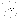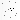## fullrun_ccd2_acisf12156_pi14-548_cl_pass1_csvfiles

#### Source 1

Data taken from csv/fullrun_ccd2_acisf12156_pi14-548_cl_pass1_tfrozen_src1.csv
X132558.68-430430.5
C/dof = 642.79/1934
Total counts = 109
Model total counts = 110.1
1 const = 0.005992 (0.001919 - 0.01398)
Model 1
Model 1
2 linx = -6.268e-05 (-0.001052 - 0.001256)
3 liny = -8.023e-05 (-0.0005338 - 0.0005123)
Model 2
Model 2
4 xc = -1.813 (-2.459 - -1.112)
5 yc = 0.1711 (-0.5496 - 0.8918)
6 norm = 0.4458 (0.3536 - 0.6245)
7 sigmax = 2.907 (2.435 - 3.611)
8 sigmay = 2.907 (= 7)
9 theta = 0 (frozen)
Model 3
Model 3
Model 3 Position (J2000) = 13 25 58.68 -43 4 30.5 (201.494498, -43.075134)
Model 3 Position (xc, yc) = (19.815407, 846.652185)data model resid

#### Source 2

Data taken from csv/fullrun_ccd2_acisf12156_pi14-548_cl_pass1_tfrozen_src2.csv
X132540.54-431057.7
C/dof = 573.11/2201
Total counts = 64
Model total counts = 66.6
1 const = 0.02928 (0.02188 - 0.0373)
Model 1
Model 1
2 linx = 0.001172 (-0.001109 - 0.003094)
3 liny = -0.0002802 (-0.002047 - 0.001407)
Model 2
Model 2
4 xc = 15.63 (>15.63)
5 yc = 15.63 (>15.63)
6 norm = 1.001e-06 (<3.028e-06)
7 sigmax = 0.977 (<0.9852)
8 sigmay = 0.977 (= 7)
9 theta = 0 (frozen)
Model 3
Model 3
Model 3 Position (J2000) = 13 25 40.54 -43 10 57.7 (201.418896, -43.182698)
Model 3 Position (xc, yc) = (424.770935, 60.229065)data model resid

#### Source 3

Data taken from csv/fullrun_ccd2_acisf12156_pi14-548_cl_pass1_tfrozen_src3.csv
X132544.43-431045.4
C/dof = 487.81/2908
Total counts = 49
Model total counts = 52.5
1 const = 0.01765 (0.01271 - 0.02276)
Model 1
Model 1
2 linx = 0.0008636 (-3.047e-05 - 0.001311)
3 liny = -0.0003054 (-0.001094 - 0.0005647)
Model 2
Model 2
4 xc = 17.99 (>17.59)
5 yc = -17.99 (indef)
6 norm = 1e-06 (<4.19e+05)
7 sigmax = 8.993 (indef)
8 sigmay = 8.993 (= 7)
9 theta = 0 (frozen)
Model 3
Model 3
Model 3 Position (J2000) = 13 25 44.43 -43 10 45.4 (201.435143, -43.179290)
Model 3 Position (xc, yc) = (338.057520, 85.057520)data model resid

#### Source 4

Data taken from csv/fullrun_ccd2_acisf12156_pi14-548_cl_pass1_tfrozen_src4.csv
X132544.65-430602.3
C/dof = 119.61/373
Total counts = 15
Model total counts = 14.0
1 const = 0.03654 (0.0215 - 0.06254)
Model 1
Model 1
2 linx = 0.005254 (-0.006669 - 0.01183)
3 liny = -0.00147 (-0.007104 - 0.006631)
Model 2
Model 2
4 xc = -6.63 (indef)
5 yc = -6.63 (indef)
6 norm = 1e-06 (<4.19e+05)
7 sigmax = 0.4144 (indef)
8 sigmay = 0.4144 (= 7)
9 theta = 0 (frozen)
Model 3
Model 3
Model 3 Position (J2000) = 13 25 44.65 -43 6 2.3 (201.436038, -43.100649)
Model 3 Position (xc, yc) = (332.523801, 660.476199)data model resid

#### Source 5

Data taken from csv/fullrun_ccd2_acisf12156_pi14-548_cl_pass1_tfrozen_src5.csv
X132528.20-430252.7
C/dof = 59.73/249
Total counts = 14
Model total counts = 13.1
1 const = 1.642e-29 (<7.475e-27)
Model 1
Model 1
2 linx = -0.008345 (-0.02627 - 0.002899)
3 liny = 0.00837 (-0.003531 - 0.0253)
Model 2
Model 2
4 xc = 0.2861 (-0.04726 - 0.5913)
5 yc = 0.4088 (0.07469 - 0.7098)
6 norm = 1.902 (0.8321 - 3.716)
7 sigmax = 0.4942 (0.3876 - 0.7216)
8 sigmay = 0.4942 (= 7)
9 theta = 0 (frozen)
Model 3
Model 3
Model 3 Position (J2000) = 13 25 28.20 -43 2 52.7 (201.367485, -43.047983)
Model 3 Position (xc, yc) = (698.581415, 1046.169059)data model resid

#### Source 6

Data taken from csv/fullrun_ccd2_acisf12156_pi14-548_cl_pass1_tfrozen_src6.csv
X132543.38-430755.7
C/dof = 134.30/890
Total counts = 13
Model total counts = 14.3
1 const = 0.01667 (0.009805 - 0.02573)
Model 1
Model 1
2 linx = 0.001548 (-0.002569 - 0.00386)
3 liny = -0.001786 (-0.003655 - 0.001145)
Model 2
Model 2
4 xc = 9.857 (>9.059)
5 yc = -9.857 (indef)
6 norm = 1e-06 (<0.4654)
7 sigmax = 0.6161 (indef)
8 sigmay = 0.6161 (= 7)
9 theta = 0 (frozen)
Model 3
Model 3
Model 3 Position (J2000) = 13 25 43.38 -43 7 55.7 (201.430755, -43.132148)
Model 3 Position (xc, yc) = (361.035407, 430.035407)data model resid